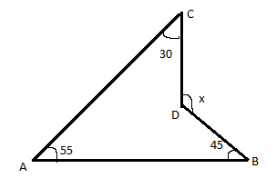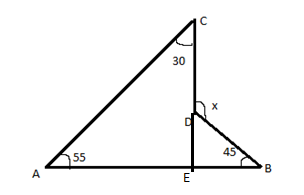QUESTION

# Find the value of x in the given figure.Hint: In order to find the value of x, first we will extend CD to cut AB at E point. Then we will use the property of the exterior angle of the triangle which states that an exterior angle of a triangle is equal to the sum of the opposite interior angles.Given angles are
$\angle ACD = {30^0} \\ \angle CAB = {55^0} \\ \angle ABD = {45^0} \\$
We have to find $\angle CBD{\text{ or x}}$
First we will extend the CD to cut AB at point E.
Now, consider $\Delta BDE,$ we have
Exterior angle as $\angle CDB$
We know that the exterior angle of a triangle is equal to the sum of the opposite interior angles.
By applying this exterior angle property, we have
$\Rightarrow \angle CDB = \angle CEB + \angle DBE$
Put the value of $\angle CDB$ as x and $\angle DBE{\text{ as 4}}{{\text{5}}^0},$ we have
$\Rightarrow x = \angle CEB + {45^0}.........(1)$
Now in $\Delta AEC,$ we have exterior angle as $\angle CED$
We know that the exterior angle of a triangle is equal to the sum of the opposite interior angles.
By applying this exterior angle property, we have
$\Rightarrow \angle CEB = \angle CAB + \angle ACE$
Substitute the value of $\Rightarrow \angle CAB{\text{ as 5}}{{\text{5}}^0}$ and $\angle ACE = {30^0},$ we get
$\Rightarrow x = {85^0} + {45^0} \\ \Rightarrow x = {130^0} \\$
Hence, the value of x is ${130^0}$ .

Note: In order to solve these types of questions, learn all the properties of triangles and the theorems related to them. Also it is important to learn about angles and parallel lines. When two lines intersect they form two pairs of opposite angles, the vertically opposite angles are equal. Also when the sum of two angles are equal then the angles are called supplementary angles.